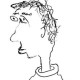## Irregular Lifts, Part I

This post motivated in part by the recent preprint of Fakhruddin, Khare, and Patrikis, and also by Matt’s number theory seminar at Chicago this week. (If you are interested in knowing what the calendar is for the Chicago number theory seminar this quarter, then that makes two of us. Actually, if you are giving a number theory seminar at Chicago this quarter, please leave a comment on this post with the day you are visiting, because several readers of this blog would be interested in finding out who is coming and what they are talking about.)

Let$\overline{\rho}: G_{\mathbf{Q}_p} \rightarrow \mathrm{GL}_n(\overline{\mathbf{F}}_p)$

be a continuous representation. We now know, by the work of Emerton-Gee, that this representation admits a lift to characteristic zero representation of regular weight which is de Rham (and is even potentially diagonalizable).

On the other hand, can it be the case that there do not exist any de Rham lifts in non-regular weight?

In the most extreme case, where we demand that all the Hodge–Tate weights are zero, then there are obstructions to lifting. In this case, the image of inertia on any lift must have finite image, but the image of inertia of$\overline{\rho}$ may already be sufficiently large to preclude this possibility. (This was exploited in the proof of Theorem 5.1 here.) So this answers the case when n = 2.

But what happens (for example) for n > 2 and HT weights = [0,…,0,1]? Or even n = 2 and replacing$\mathbf{Q}_p$ by a finite extension K? The first remark is that even when the residual image lands inside the Borel, there will certainly be obstructions to finding lifts inside the Borel, which means that inductive arguments will not be sufficient. On the other hand, this definitely smells like a tractable problem.

I offer an Aperol Spritz to an answer to this problem — let me do so even in the constrained version in weight [0,0,1] and$K = \mathbf{Q}_p.$

This entry was posted in Mathematics and tagged , , , , , , , , . Bookmark the permalink.

### 6 Responses to Irregular Lifts, Part I

1.arithmetica says:

As requested: I’m speaking on October 30th, about completed cohomology.

2.TG says:

10/23 Tony Feng
10/30 David Hansen
11/6 Alexander Smith
11/13 Jessica Fintzen
11/27 Will Sawin
12/04 Chi-Yun Hsu

3.PC says:

Some rule of thumb computation suggest that de Rham liftings of representations of $G_{{\mathbf Q}_p}$ with HT weights $0,0,1$ come in (non-empty) varieties of dimension $2$ (ignoring twists by an unramified character). Since the mod $p$ representation has a lifting in characteristic $0$, the space of such liftings $V$ should be of dimension $10$ (or just $9$ if one ignores unramified twists). The theory of $(\varphi,\Gamma)$-modules (or Fontaine’s extension of Sen’s theory) produces a module $D_{\mathrm dif}^+$ of rank $3$ over $F_n[[t]]$ for $n$ big (and $F_n$ is the $n$-th layer in the cyclotomic tower) with a connexion $\nabla$ (with $\nabla t=t$) and $V$ is de Rham if and only if the connexion is trivial (after inverting $t$). So, if all weights are $0$, for example, this is equivalent to $\nabla=0$ on $D_{\mathrm Sen}=D_{\mathrm dif}^+/t$, which gives $9$ conditions and explains why de Rham representations with HT weights $0,0,0$ are isolated. Now, in the case $0,0,1$, you need $\nabla$ to be of trace and rank $1$ on $D_{\mathrm Sen}$ which gives you $4$ parameters (a line in $P^2$, $3$ vectors in this line, and a relation implying that the trace is $1$), and each such $\nabla$ produces a plane $W$ on which $\nabla$ is trivial, and a line $K_n[[t]] f$ with $\nabla f-f\in tD_{\mathrm dif}^+$ and the connexion is trivial if and only if $\nabla f-f$ has no component in $tW/t^2W$, which gives $2$ extra conditions. So you are left with $2$-dimensional families (if you do the same thing with HT weights $0,1,2$, you end up with $3$-dimensional families). If one wants to turn the above into a rigorous argument, I am afraid that some work is needed…. (One needs facts about the map sending a representation to its Sen operator which are maybe not in the literature.)

•galoisrepresentations says:

I certainly agree with the numerology here, having recently done a similar calculation — at a weight corresponding to a dominant cocharacter$\lambda,$ the stabilizer of this cocharacter inside the Weyl group will be of the form$S_{a_1} \times S_{a_2} \times \ldots S_{a_k} \subset S_n$

with$\sum a_i = n$ giving rise to a Levi subgroup$\mathrm{GL}(a_1) \times \ldots \mathrm{GL}(a_k),$ and then the “expected” dimension of the deformation ring with fixed determinant should be$\displaystyle{\mathrm{dim}(B(n)) - \sum_{i=1}^{k} \mathrm{dim}(B(a_i))}$$\displaystyle{ = \frac{n(n+1)}{2} - \sum_{i=1}^{k} \frac{a_i(a_i+1)}{2}}$$\displaystyle{= \frac{n(n-1)}{2} - \sum_{i=1}^{k} \frac{a_i(a_i-1)}{2},}$

which (for 3 = 2 + 1 = 1 + 1 + 1) gives 0, 2, and 3 respectively. And this number certainly positive unless one is in trivial weight. What I’m not sure of, however, if you are arguing that this is a *heuristic* for the existence of lifts or a *strategy* for proving so. The heuristic doesn’t entirely convince me — in part because the heuristic still *somewhat* suggests there should still be lifts of weight zero (because 0 > -1). If you are saying this is a strategy, then the fact that these Sen maps are only locally analytic certainly makes me nervous, combined with the fact that p-adic balls are not projective spaces so if you want to impose A conditions in B dimensions then you still have work to do when B > A to show there are solutions… of course you know this better than me, so you might have a better feeling for how worried one should be.

4.PC says:

That was more a heuristic than a strategy, but I agree that your observation that 0 > -1 makes this heuristic somewhat shaky…